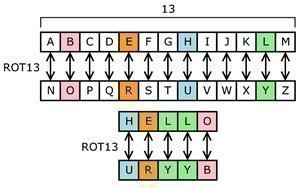•• # Substitution and Transposition Ciphers

Substitution and transposition ciphers are two categories of ciphers used in classical cryptography. Substitution and transposition differ in how chunks of the message are handled by the encryption process.

## Substitution ciphers

Substitution ciphers encrypt plaintext by changing the plaintext one piece at a time.

The Caesar Cipher was an early substitution cipher. In the Caesar Cipher, each character is shifted three places up. Therefore, A becomes D and B becomes E, etc…

This table shows “VOYAGER” being encrypted with the Caesar substitution cipher:Plaintext Key Ciphertext V O Y A G E R +3 +3 +3 +3 +3 +3 +3 Y R B D J H U

A more complex substitution cipher would be created if, instead of incrementing each character by three, we used a more complex key. This table shows a simple substitution cipher with a key of “123”.

 Plaintext Key Ciphertext V O Y A G E R +1 +2 +3 +1 +2 +3 +1 W Q B B I H S

An even more complex substitution cipher can be made by having each character of the alphabet correspond to a different letter of the alphabet, without a set pattern.

 Plaintext Key A B C D E F G H I J K L M N O P Q R S T U V W X Y Z T O E U N Z I A G X P Q Y R H V S M D F C J W B K L

Using this substitution cipher to encrypt VOYAGER would give us these results:

 Plaintext Ciphertext V O Y A G E R J H K T X N M

The Vernam Cipher, or one time pad, is a simple substitution cipher where the key length equals the message length.

ROT-1 is a simple substitution cipher used to encode messages on Usenet.

## Transposition ciphers

Transposition ciphers encrypt plaintext by moving small pieces of the message around. Anagrams are a primitive transposition cipher.

This table shows “VOYAGER” being encrypted with a primitive transposition cipher where every two letters are switched with each other:

 V O Y A G E R O V A Y E G R

## Substitution and transposition ciphers in modern times

Modern cryptanalysis makes simple substitution and transposition ciphers obsolete.

However, these techniques remain useful for understanding cryptography and the workings of more complex modern ciphers.

1. #### saurabh h

15 November, 2016 at 7:49 am

It hepled a lot.

2. #### PeaceMan

10 September, 2015 at 8:29 am

Thanks!